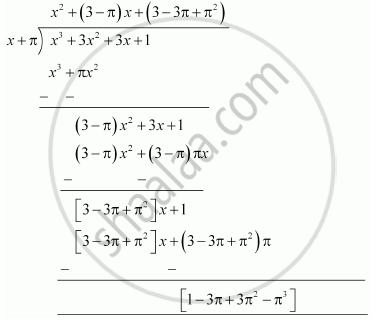# Find the remainder when x^3 + 3x^2 + 3x + 1 is divided by x + π. - Mathematics

Find the remainder when x3 + 3x2 + 3x + 1 is divided by x + π.

#### Solution 1

Let p(x) = x3 + 3x2 + 3x + 1

x + π = 0 ⇒ x = -π

∴ Remainder = (-π)3 + 3(-π)2 - 3(-π) + 1

= -π3 + 3π2 - 3π + 1

Therefore, the remainder is -π3 + 3π2 - 3π + 1.

#### Solution 2

By long division,Therefore, the remainder is -π3 + 3π2 - 3π + 1.

Concept: Remainder Theorem
Is there an error in this question or solution?

#### APPEARS IN

NCERT Class 9 Maths
Chapter 2 Polynomials
Exercise 2.3 | Q 1.4 | Page 40